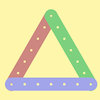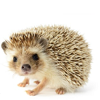#### You may also like### I'm Eight

Find a great variety of ways of asking questions which make 8.### Let's Investigate Triangles

Vincent and Tara are making triangles with the class construction set. They have a pile of strips of different lengths. How many different triangles can they make?### Noah

Noah saw 12 legs walk by into the Ark. How many creatures did he see?

# Two Numbers Under the Microscope

##### Age 5 to 7Challenge Level

Well done to everybody who had a go at this activity. We had lots of solutions sent in from the pupils at Halstead Preparatory School in the UK, so thank you all for sending us your ideas!

Lots of children noticed that adding two odd numbers together gives an answer that is an even number. Bea from Halstead Preparatory School had a go at explaning why this is true. She said:

2 odd numbers always to add up to an even.
Odd numbers are one short of an even number. They get that one from the other odd number.

This is a great explanation, Bea - well done!

Pieter from the British International School in Istanbul in Turkey explained the pattern with similar reasoning:

An odd number actually is an "even number plus 1" (or minus 1). So if you add two odd numbers, both "1"s from both numbers together make a "2", which is an even number; and the remaining part of both numbers were even anyway, so the total is always even.

Well done, Pieter!

James from St. John's School in Northwood, England investigated what would happen if we put more numbers under the microscope:

They always add up to an even number because if you add together the even numbers that are one less than each number you'll have an even number. Then if you add together the two that are left you get an even number. If you do this with three odd numbers it would give you an odd number because you'll have three left to add together. If you add up an even number of odd numbers you get an even number but if you have an odd number of odd numbers you get an odd number.

Good ideas, James! I wonder if we could draw a picture to represent what happens when we add odd numbers together?